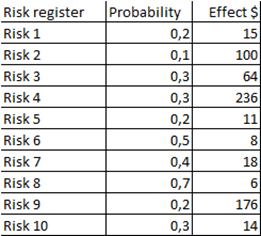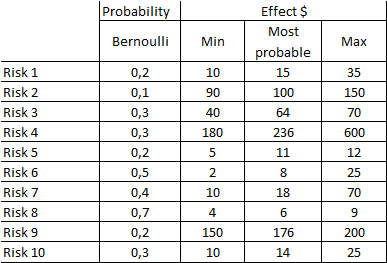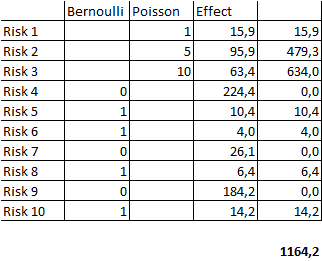## What is a risk register why you DO NOT need it

Let’s illustrate the type of problems typical risk registers create and why the use of qualitative or scoring risk registers use should be avoided by management and not accepted by the Boards.Imagine the company has 10 risks in its corporate or project risk register. Board wants to know how these 10 risks affect the objectives and to know the aggregate risk exposure.Each risk exposure is usually calculated by multiplying probability and effect and then risks are summed to determine the aggregate risk exposure. This, seemingly simple process, leads to very misleading and incorrect conclusions.

Let’s investigate a better way to measure risks. Risk 1 can occur with 20% probability. So, there are 2 possible scenarios: Risk 1 occurs (with probability 20%) and Risk 1 does not occur (with probability 80%).

If Risk 1 occurs the effect is 15\$. If Risk 1 does not occur the effect is 0\$. With the exception of some risks (market for example), there are no in-between cases, risk either happens or it doesn’t. The previous risk exposure Probability Х Effect = 3\$ is totally misleading.

Alex has created a short bootcamp designed to help companies implement quantitative risk management. Imagine saving the company so much money that investing in risk management competencies and resources becomes a no brainer for the executives. That's exactly what Alex Sidorenko did at a global \$10B chemical company and he has been kind enough to share his top tips and lessons learned with you each week. Sign up now!

Here are the reasons:

• We need to consider both scenarios (risk occurs and does not occur) and effects of scenario separately
• We need to consider dependency between risks, it is very unlikely that all 10 risks occur simultaneously or completely independently. Simple addition only works for calculating expected losses some of the time. A simulation is required to aggregate risks, it will be covered in more detail in Modules 3 and 4.
• Some risks may happen more than once per year, so probability no longer works and needs to be replaced by frequency.
• Effect from any one risk is actually a range of potential values. Rarely when risk occurs there is a certain fixed effect on the business. Effects need to be represented as probability distributions not single values.

Let’s compare the risk register above to a better alternative, still very simplified for the purposes of illustration only:Because risks don’t occur on average, probabilities are better represented as Bernoulli distributions and they either occur or they don’t. Effects are also uncertain and are best represented by another distribution, this time continuous, PERT just for illustration purposes. PERT has a minimum, most likely and maximum values. We used our original effect values as mode. To calculate the total, we run a simulation.The original sum of the risks was 160. We can now see sum of the risks is actually a range from 0 to 610. In fact, there is 54% chance that the total risk for the year will be above original 160. 95% of the time (also sometimes called VaR) total risk exposure will be below 490. Pretending that total risk is 160 is actually bad decision making and will mean risks most likely will not be mitigated properly and underfunded.The above example is also not very realistic because it is assuming that every risk can only happen once per year.For illustration purposes we replaced the distribution for the first 3 risks to occur more than once per year, it is now 1, 5 and 10 times per year accordingly. This seemingly simple change in assumptions has a significant impact on the overall risk exposure.When we compare a scenario where risks 1, 2 and 3 can occur multiple times per year, the risk exposure went from 0 to 610 to 17 to 3350 and the risk profile looks very different. Pink in the diagram is a single risk per year, green is multiple risks 1,2 and 3. The chance above original 160 risk exposure is now 100%. In fact, 95% of the time the risk exposure will be below 1919. Let that sink in, the risk exposure is 10X originally thought.That is not the end, however. Previously we made the assumption that all risks are independent and are not correlated to one another. In reality, some risks may be codependent, and if one occurs, the other is more likely to occur as well. Green in the diagram is the previous uncorrelated risk, the pink is the risk profile given correlation. Given correlation, 95% of the time the risk exposure will be below 2440, that’s almost 500 higher than without correlation.

### Now cast your mind back to the original risk total of 160, that’s 15X difference compared to a more accurate risk estimate. All we did was to use the better methodology.

That’s just the risk analysis part of the risk register, the mitigation, risk owner and other columns have as much issues if not more. The overall theme of the article is DO NOT use risk registers, but if you ever needed one, keep in mind:

• total risk is itself not that important, it is the effect sum of correlated risks have on a decision or objective are important
• total risk can be low or high, we don’t know whether this is good or bad unless some measure of tolerance is determined, maybe a lot of risks is good for the company

## 4 thoughts on “What is a risk register why you DO NOT need it”

1.Norman Marks says:

Alex, the solution you suggest is better but:
1. It does not show the effect on objectives – and more than one objective might be affected
2. It addresses one source of risk at a time
3. It ignores the reason for taking risk, the reward

1.Alex Sidorenko says:

1. The risk register is using effect instead of typical consequences specifically to address that point
2. Not really, the scope of the article is about something else
3. Not really, the scope of the article is about something else

2.Mike Shopland says:
1.Alex Sidorenko says: